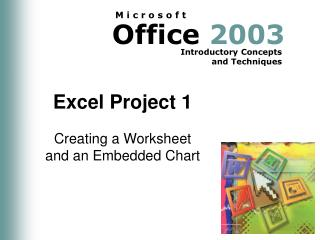DownloadDownload PresentationExcel Project 1

# Excel Project 1

Télécharger la présentation## Excel Project 1

- - - - - - - - - - - - - - - - - - - - - - - - - - - E N D - - - - - - - - - - - - - - - - - - - - - - - - - - -
##### Presentation Transcript

1. Excel Project 1 Creating a Worksheet and an Embedded Chart

2. Introduction to Excel • Microsoft Office Excel is a powerful spreadsheet program that allows users to organize data, complete calculations, graph data, develop professional looking reports and more

3. Four main components of Excel • Worksheets: Allow users to enter, calculate, manipulate, and analyze data (numbers and text). Worksheet means the same as Spreadsheet. • Charts: Excel can draw a variety of charts.

4. Four main components of Excel • Lists: Lists organize and store data. You can sort, search, and select data. • Web Support Allows users to save Excel worksheets in html format

5. How Excel Works • When Excel starts it creates a new blank workbook, called book1. The workbook is like a notebook. Inside the workbook are sheets, which are referred as worksheet. • The worksheet is organized into a rectangular grid.

6. Terminology • Column Heading: A column letter above the grid identifies each column • Row Heading: A row number on the left side of the grid identifies each row • Cell: is the basic unit of a worksheet. It is where a column and row meet. • Each worksheet in a workbook has 256 columns and 65536 rows

7. Terminology • Cell Reference: Identifies each cell uniquely. It is the letter from the column heading and the number from the row heading. • Active cell: the cell that you are currently in • Formula Bar: As you typed Excel displays the entry in the formula bar

8. Excel Project 1 Complete